Documentation

## Speech Command Recognition Using Deep Learning

This example shows how to train a simple deep learning model that detects the presence of speech commands in audio. The example uses the Speech Commands Dataset  to train a convolutional neural network to recognize a given set of commands.

To run the example, you must first download the data set. If you do not want to download the data set or train the network, then you can load a pretrained network by opening this example in MATLAB® and typing `load('commandNet.mat')` at the command line. After loading the network, go directly to the last section of this example, Detect Commands Using Streaming Audio from Microphone.

### Load Speech Commands Data Set

Download the data set from https://storage.googleapis.com/download.tensorflow.org/data/speech_commands_v0.01.tar.gz and extract the downloaded file. Set `datafolder` to the location of the data. Use `audioDatastore` to create a datastore that contains the file names and the corresponding labels. Use the folder names as the label source. Specify the read method to read the entire audio file. Create a copy of the datastore for later use.

```datafolder = PathToDatabase; ads = audioDatastore(datafolder, ... 'IncludeSubfolders',true, ... 'FileExtensions','.wav', ... 'LabelSource','foldernames') ads0 = copy(ads); ```
```ads = audioDatastore with properties: Files: { ' ...\datasets\google_speech\_background_noise_\doing_the_dishes.wav'; ' ...\datasets\google_speech\_background_noise_\dude_miaowing.wav'; ' ...\datasets\google_speech\_background_noise_\exercise_bike.wav' ... and 64724 more } Labels: [_background_noise_; _background_noise_; _background_noise_ ... and 64724 more categorical] AlternateFileSystemRoots: {} OutputDataType: 'double' ```

### Choose Words to Recognize

Specify the words that you want your model to recognize as commands. Label all words that are not commands as `unknown`. Labeling words that are not commands as `unknown` creates a group of words that approximates the distribution of all words other than the commands. The network uses this group to learn the difference between commands and all other words.

To reduce the class imbalance between the known and unknown words and speed up processing, only include a fraction `includeFraction` of the unknown words in the training set. Do not include the longer files with background noise in the training set yet. Background noise will be added in a separate step later.

Use `subset(ads,indices)` to create a datastore that contains only the files and labels indexed by `indices`. Reduce the datastore `ads` so that it contains only the commands and the subset of unknown words. Count the number of examples belonging to each class.

```commands = categorical(["yes","no","up","down","left","right","on","off","stop","go"]); isCommand = ismember(ads.Labels,commands); isUnknown = ~ismember(ads.Labels,[commands,"_background_noise_"]); includeFraction = 0.2; mask = rand(numel(ads.Labels),1) < includeFraction; isUnknown = isUnknown & mask; ads.Labels(isUnknown) = categorical("unknown"); ads = subset(ads,isCommand|isUnknown); countEachLabel(ads) ```
```ans = 11×2 table Label Count _______ _____ down 2359 go 2372 left 2353 no 2375 off 2357 on 2367 right 2367 stop 2380 unknown 8294 up 2375 yes 2377 ```

### Split Data into Training, Validation, and Test Sets

The data set folder contains text files, which list the audio files to be used as the validation and test sets. These predefined validation and test sets do not contain utterances of the same word by the same person, so it is better to use these predefined sets than to select a random subset of the whole data set. Use the supporting function `splitData` to split the datastore into training, validation, and test sets based on the list of validation and test files located in the data set folder.

Because this example trains a single network, it only uses the validation set and not the test set to evaluate the trained model. If you train many networks and choose the network with the highest validation accuracy as your final network, then you can use the test set to evaluate the final network.

```[adsTrain,adsValidation,adsTest] = splitData(ads,datafolder); ```

### Compute Speech Spectrograms

To prepare the data for efficient training of a convolutional neural network, convert the speech waveforms to log-mel spectrograms.

Define the parameters of the spectrogram calculation. `segmentDuration` is the duration of each speech clip (in seconds). `frameDuration` is the duration of each frame for spectrogram calculation. `hopDuration` is the time step between each column of the spectrogram. `numBands` is the number of log-mel filters and equals the height of each spectrogram.

```segmentDuration = 1; frameDuration = 0.025; hopDuration = 0.010; numBands = 40; ```

Compute the spectrograms for the training, validation, and test sets by using the supporting function `speechSpectrograms`. The `speechSpectrograms` function uses `designAuditoryFilterBank` for the log-mel spectrogram calculations. To obtain data with a smoother distribution, take the logarithm of the spectrograms using a small offset `epsil`.

```epsil = 1e-6; XTrain = speechSpectrograms(adsTrain,segmentDuration,frameDuration,hopDuration,numBands); XTrain = log10(XTrain + epsil); XValidation = speechSpectrograms(adsValidation,segmentDuration,frameDuration,hopDuration,numBands); XValidation = log10(XValidation + epsil); XTest = speechSpectrograms(adsTest,segmentDuration,frameDuration,hopDuration,numBands); XTest = log10(XTest + epsil); YTrain = adsTrain.Labels; YValidation = adsValidation.Labels; YTest = adsTest.Labels; ```
```Computing speech spectrograms... Processed 1000 files out of 25128 Processed 2000 files out of 25128 Processed 3000 files out of 25128 Processed 4000 files out of 25128 Processed 5000 files out of 25128 Processed 6000 files out of 25128 Processed 7000 files out of 25128 Processed 8000 files out of 25128 Processed 9000 files out of 25128 Processed 10000 files out of 25128 Processed 11000 files out of 25128 Processed 12000 files out of 25128 Processed 13000 files out of 25128 Processed 14000 files out of 25128 Processed 15000 files out of 25128 Processed 16000 files out of 25128 Processed 17000 files out of 25128 Processed 18000 files out of 25128 Processed 19000 files out of 25128 Processed 20000 files out of 25128 Processed 21000 files out of 25128 Processed 22000 files out of 25128 Processed 23000 files out of 25128 Processed 24000 files out of 25128 Processed 25000 files out of 25128 ...done Computing speech spectrograms... Processed 1000 files out of 3391 Processed 2000 files out of 3391 Processed 3000 files out of 3391 ...done Computing speech spectrograms... Processed 1000 files out of 3457 Processed 2000 files out of 3457 Processed 3000 files out of 3457 ...done ```

### Visualize Data

Plot the waveforms and spectrograms of a few training examples. Play the corresponding audio clips.

```specMin = min(XTrain(:)); specMax = max(XTrain(:)); idx = randperm(size(XTrain,4),3); figure('Units','normalized','Position',[0.2 0.2 0.6 0.6]); for i = 1:3 [x,fs] = audioread(adsTrain.Files{idx(i)}); subplot(2,3,i) plot(x) axis tight title(string(adsTrain.Labels(idx(i)))) subplot(2,3,i+3) spect = XTrain(:,:,1,idx(i)); pcolor(spect) caxis([specMin+2 specMax]) shading flat sound(x,fs) pause(2) end ```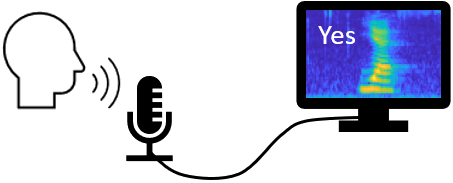Training neural networks is easiest when the inputs to the network have a reasonably smooth distribution and are normalized. To check that the data distribution is smooth, plot a histogram of the pixel values of the training data.

```figure histogram(XTrain,'EdgeColor','none','Normalization','pdf') axis tight ax = gca; ax.YScale = 'log'; xlabel("Input Pixel Value") ylabel("Probability Density") ```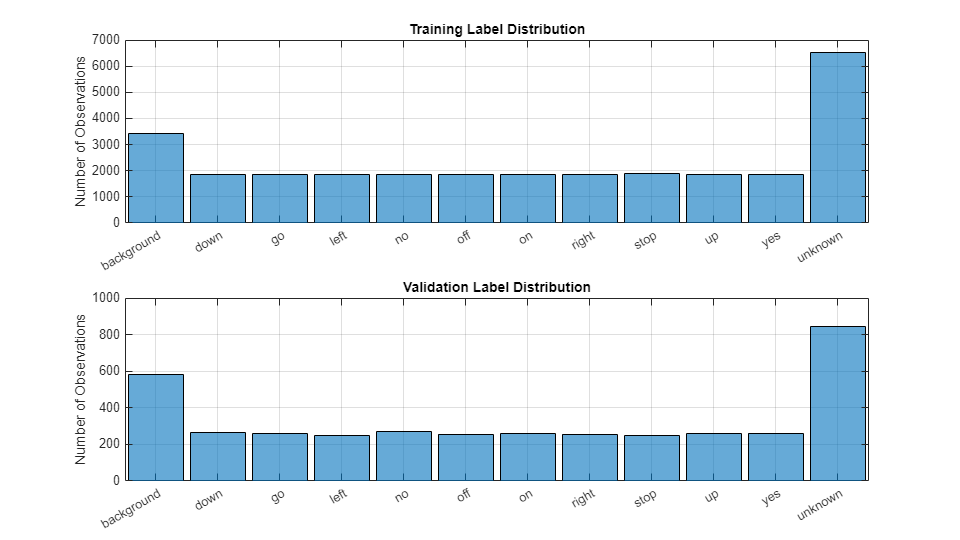The network must be able not only to recognize different spoken words but also to detect if the input contains silence or background noise.

Use the audio files in the `_background_noise`_ folder to create samples of one-second clips of background noise. Create an equal number of background clips from each background noise file. You can also create your own recordings of background noise and add them to the `_background_noise`_ folder. To calculate `numBkgClips` spectrograms of background clips taken from the audio files in the `adsBkg` datastore, use the supporting function `backgroundSpectrograms`. Before calculating the spectrograms, the function rescales each audio clip with a factor sampled from a log-uniform distribution in the range given by `volumeRange`.

Create 4,000 background clips and rescale each by a number between `1e-4` and `1`. `XBkg` contains spectrograms of background noise with volumes ranging from practically silent to loud.

```adsBkg = subset(ads0,ads0.Labels=="_background_noise_"); numBkgClips = 4000; volumeRange = [1e-4,1]; XBkg = backgroundSpectrograms(adsBkg,numBkgClips,volumeRange,segmentDuration,frameDuration,hopDuration,numBands); XBkg = log10(XBkg + epsil); ```
```Computing background spectrograms... Processed 1000 background clips out of 4000 Processed 2000 background clips out of 4000 Processed 3000 background clips out of 4000 Processed 4000 background clips out of 4000 ...done ```

Split the spectrograms of background noise between the training, validation, and test sets. Because the `_background_noise`_ folder contains only about five and a half minutes of background noise, the background samples in the different data sets are highly correlated. To increase the variation in the background noise, you can create your own background files and add them to the folder. To increase the robustness of the network to noise, you can also try mixing background noise into the speech files.

```numTrainBkg = floor(0.8*numBkgClips); numValidationBkg = floor(0.1*numBkgClips); numTestBkg = floor(0.1*numBkgClips); XTrain(:,:,:,end+1:end+numTrainBkg) = XBkg(:,:,:,1:numTrainBkg); XBkg(:,:,:,1:numTrainBkg) = []; YTrain(end+1:end+numTrainBkg) = "background"; XValidation(:,:,:,end+1:end+numValidationBkg) = XBkg(:,:,:,1:numValidationBkg); XBkg(:,:,:,1:numValidationBkg) = []; YValidation(end+1:end+numValidationBkg) = "background"; XTest(:,:,:,end+1:end+numTestBkg) = XBkg(:,:,:,1: numTestBkg); clear XBkg; YTest(end+1:end+numTestBkg) = "background"; YTrain = removecats(YTrain); YValidation = removecats(YValidation); YTest = removecats(YTest); ```

Plot the distribution of the different class labels in the training and validation sets. The test set has a very similar distribution to the validation set.

```figure('Units','normalized','Position',[0.2 0.2 0.5 0.5]); subplot(2,1,1) histogram(YTrain) title("Training Label Distribution") subplot(2,1,2) histogram(YValidation) title("Validation Label Distribution") ```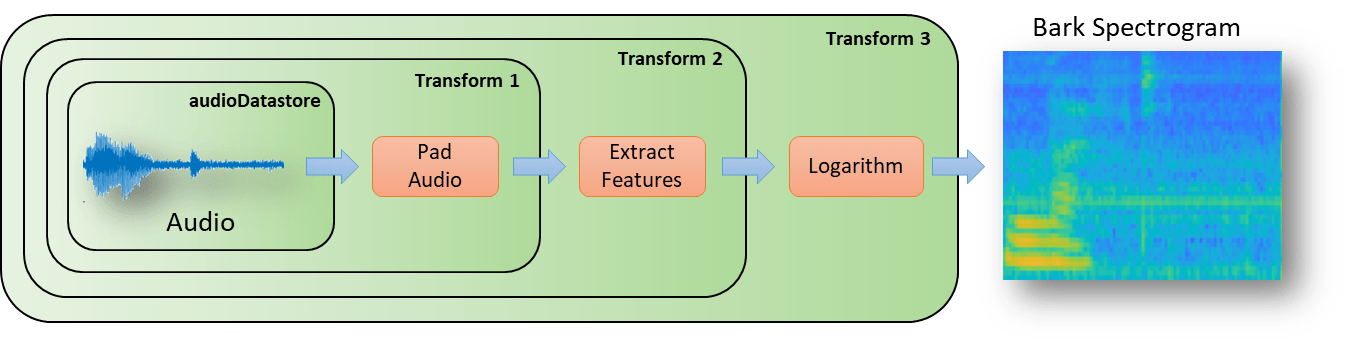Create an augmented image datastore for automatic augmentation and resizing of the spectrograms. Translate the spectrogram randomly up to 10 frames (100 ms) forwards or backwards in time, and scale the spectrograms along the time axis up or down by 20 percent. Augmenting the data can increase the effective size of the training data and help prevent the network from overfitting. The augmented image datastore creates augmented images in real time during training and inputs them to the network. No augmented spectrograms are saved in memory.

```sz = size(XTrain); specSize = sz(1:2); imageSize = [specSize 1]; augmenter = imageDataAugmenter( ... 'RandXTranslation',[-10 10], ... 'RandXScale',[0.8 1.2], ... 'FillValue',log10(epsil)); augimdsTrain = augmentedImageDatastore(imageSize,XTrain,YTrain, ... 'DataAugmentation',augmenter); ```

### Define Neural Network Architecture

Create a simple network architecture as an array of layers. Use convolutional and batch normalization layers, and downsample the feature maps "spatially" (that is, in time and frequency) using max pooling layers. Add a final max pooling layer that pools the input feature map globally over time. This enforces (approximate) time-translation invariance in the input spectrograms, allowing the network to perform the same classification independent of the exact position of the speech in time. Global pooling also significantly reduces the number of parameters in the final fully connected layer. To reduce the possibility of the network memorizing specific features of the training data, add a small amount of dropout to the input to the last fully connected layer.

The network is small, as it has only five convolutional layers with few filters. `numF` controls the number of filters in the convolutional layers. To increase the accuracy of the network, try increasing the network depth by adding identical blocks of convolutional, batch normalization, and ReLU layers. You can also try increasing the number of convolutional filters by increasing `numF`.

Use a weighted cross entropy classification loss. `weightedClassificationLayer(classWeights)` creates a custom classification layer that calculates the cross entropy loss with observations weighted by `classWeights`. Specify the class weights in the same order as the classes appear in `categories(YTrain)`. To give each class equal total weight in the loss, use class weights that are inversely proportional to the number of training examples in each class. When using the Adam optimizer to train the network, the training algorithm is independent of the overall normalization of the class weights.

```classWeights = 1./countcats(YTrain); classWeights = classWeights'/mean(classWeights); numClasses = numel(categories(YTrain)); timePoolSize = ceil(imageSize(2)/8); dropoutProb = 0.2; numF = 12; layers = [ imageInputLayer(imageSize) convolution2dLayer(3,numF,'Padding','same') batchNormalizationLayer reluLayer maxPooling2dLayer(3,'Stride',2,'Padding','same') convolution2dLayer(3,2*numF,'Padding','same') batchNormalizationLayer reluLayer maxPooling2dLayer(3,'Stride',2,'Padding','same') convolution2dLayer(3,4*numF,'Padding','same') batchNormalizationLayer reluLayer maxPooling2dLayer(3,'Stride',2,'Padding','same') convolution2dLayer(3,4*numF,'Padding','same') batchNormalizationLayer reluLayer convolution2dLayer(3,4*numF,'Padding','same') batchNormalizationLayer reluLayer maxPooling2dLayer([1 timePoolSize]) dropoutLayer(dropoutProb) fullyConnectedLayer(numClasses) softmaxLayer weightedClassificationLayer(classWeights)]; ```

### Train Network

Specify the training options. Use the Adam optimizer with a mini-batch size of 128. Train for 25 epochs and reduce the learning rate by a factor of 10 after 20 epochs.

```miniBatchSize = 128; validationFrequency = floor(numel(YTrain)/miniBatchSize); options = trainingOptions('adam', ... 'InitialLearnRate',3e-4, ... 'MaxEpochs',25, ... 'MiniBatchSize',miniBatchSize, ... 'Shuffle','every-epoch', ... 'Plots','training-progress', ... 'Verbose',false, ... 'ValidationData',{XValidation,YValidation}, ... 'ValidationFrequency',validationFrequency, ... 'LearnRateSchedule','piecewise', ... 'LearnRateDropFactor',0.1, ... 'LearnRateDropPeriod',20); ```

Train the network. If you do not have a GPU, then training the network can take time. To load a pretrained network instead of training a network from scratch, set `doTraining` to `false`.

```doTraining = true; if doTraining trainedNet = trainNetwork(augimdsTrain,layers,options); else load('commandNet.mat','trainedNet'); end ```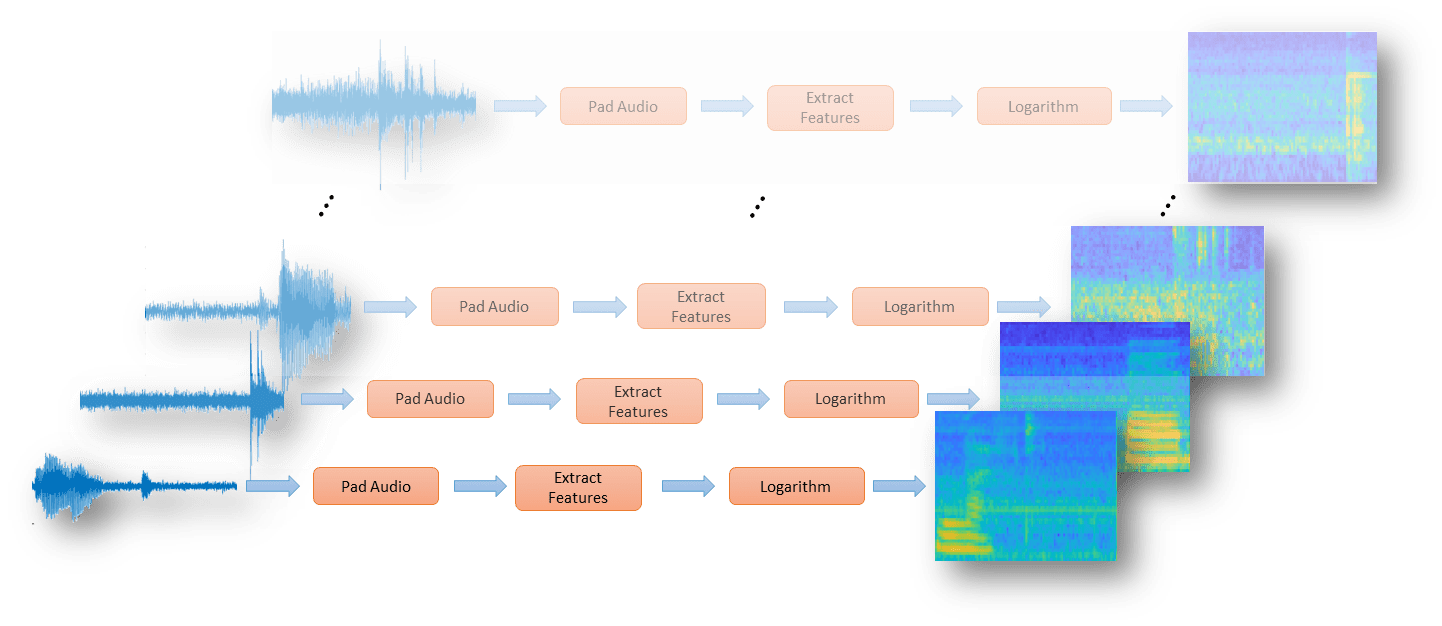### Evaluate Trained Network

Calculate the final accuracy of the network on the training set (without data augmentation) and validation set. The network is very accurate on this data set. However, the training, validation, and test data all have similar distributions that do not necessarily reflect real-world environments. This limitation particularly applies to the `unknown` category, which contains utterances of only a small number of words.

```YValPred = classify(trainedNet,XValidation); validationError = mean(YValPred ~= YValidation); YTrainPred = classify(trainedNet,XTrain); trainError = mean(YTrainPred ~= YTrain); disp("Training error: " + trainError*100 + "%") disp("Validation error: " + validationError*100 + "%") ```
```Training error: 4.0419% Validation error: 6.4099% ```

Plot the confusion matrix. Display the precision and recall for each class by using column and row summaries. Sort the classes of the confusion matrix. The largest confusion is between unknown words and commands, up and off, down and no, and go and no.

```figure('Units','normalized','Position',[0.2 0.2 0.5 0.5]); cm = confusionchart(YValidation,YValPred); cm.Title = 'Confusion Matrix for Validation Data'; cm.ColumnSummary = 'column-normalized'; cm.RowSummary = 'row-normalized'; sortClasses(cm, [commands,"unknown","background"]) ```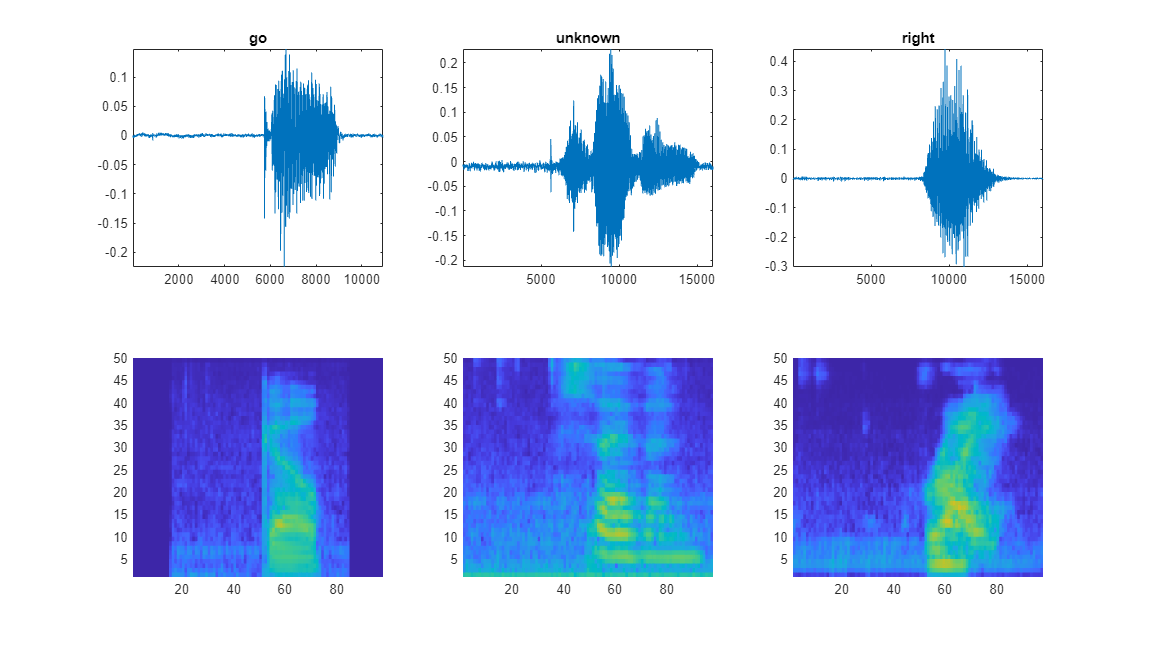When working on applications with constrained hardware resources such as mobile applications, consider the limitations on available memory and computational resources. Compute the total size of the network in kilobytes and test its prediction speed when using a CPU. The prediction time is the time for classifying a single input image. If you input multiple images to the network, these can be classified simultaneously, leading to shorter prediction times per image. When classifying streaming audio, however, the single-image prediction time is the most relevant.

```info = whos('trainedNet'); disp("Network size: " + info.bytes/1024 + " kB") for i=1:100 x = randn(imageSize); tic [YPredicted,probs] = classify(trainedNet,x,"ExecutionEnvironment",'cpu'); time(i) = toc; end disp("Single-image prediction time on CPU: " + mean(time(11:end))*1000 + " ms") ```
```Network size: 285.2109 kB Single-image prediction time on CPU: 1.9703 ms ```

### Detect Commands Using Streaming Audio from Microphone

Test your newly trained command detection network on streaming audio from your microphone. If you have not trained a network, then type `load('commandNet.mat')` at the command line to load a pretrained network and the parameters required to classify live, streaming audio. Try saying one of the commands, for example, yes, no, or stop. Then, try saying one of the unknown words such as Marvin, Sheila, bed, house, cat, bird, or any number from zero to nine.

Specify the audio sampling rate and classification rate in Hz and create an audio device reader that can read audio from your microphone.

```fs = 16e3; classificationRate = 20; audioIn = audioDeviceReader('SampleRate',fs, ... 'SamplesPerFrame',floor(fs/classificationRate)); ```

Specify parameters for the streaming spectrogram computations and initialize a buffer for the audio. Extract the classification labels of the network. Initialize buffers of half a second for the labels and classification probabilities of the streaming audio. Use these buffers to compare the classification results over a longer period of time and by that build 'agreement' over when a command is detected.

```frameLength = floor(frameDuration*fs); hopLength = floor(hopDuration*fs); waveBuffer = zeros([fs,1]); labels = trainedNet.Layers(end).Classes; YBuffer(1:classificationRate/2) = categorical("background"); probBuffer = zeros([numel(labels),classificationRate/2]); ```

Create a figure and detect commands as long as the created figure exists. To stop the live detection, simply close the figure.

```h = figure('Units','normalized','Position',[0.2 0.1 0.6 0.8]); filterBank = designAuditoryFilterBank(fs,'FrequencyScale','bark',... 'FFTLength',512,... 'NumBands',numBands,... 'FrequencyRange',[50,7000]); while ishandle(h) % Extract audio samples from the audio device and add the samples to % the buffer. x = audioIn(); waveBuffer(1:end-numel(x)) = waveBuffer(numel(x)+1:end); waveBuffer(end-numel(x)+1:end) = x; % Compute the spectrogram of the latest audio samples. [~,~,~,spec] = spectrogram(waveBuffer,hann(frameLength,'periodic'),frameLength - hopLength,512,'onesided'); spec = filterBank * spec; spec = log10(spec + epsil); % Classify the current spectrogram, save the label to the label buffer, % and save the predicted probabilities to the probability buffer. [YPredicted,probs] = classify(trainedNet,spec,'ExecutionEnvironment','cpu'); YBuffer(1:end-1)= YBuffer(2:end); YBuffer(end) = YPredicted; probBuffer(:,1:end-1) = probBuffer(:,2:end); probBuffer(:,end) = probs'; % Plot the current waveform and spectrogram. subplot(2,1,1); plot(waveBuffer) axis tight ylim([-0.2,0.2]) subplot(2,1,2) pcolor(spec) caxis([specMin+2 specMax]) shading flat % Now do the actual command detection by performing a very simple % thresholding operation. Declare a detection and display it in the % figure title if all of the following hold: % 1) The most common label is not |background|. % 2) At least |countThreshold| of the latest frame labels agree. % 3) The maximum predicted probability of the predicted label is at % least |probThreshold|. Otherwise, do not declare a detection. [YMode,count] = mode(YBuffer); countThreshold = ceil(classificationRate*0.2); maxProb = max(probBuffer(labels == YMode,:)); probThreshold = 0.7; subplot(2,1,1); if YMode == "background" || count<countThreshold || maxProb < probThreshold title(" ") else title(string(YMode),'FontSize',20) end drawnow end ```